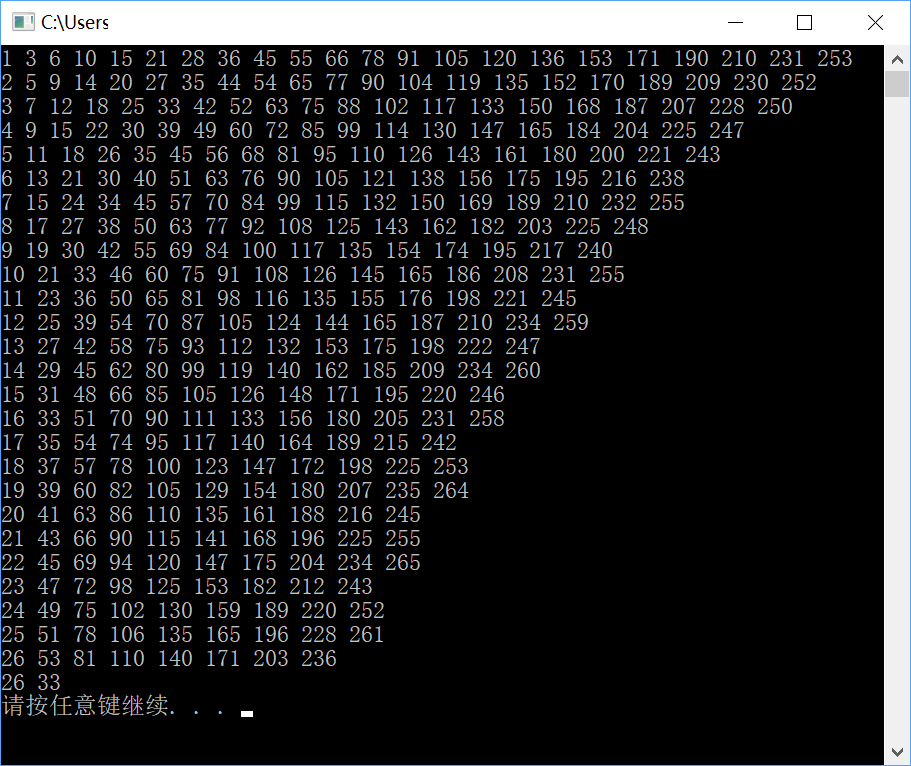###### 力～元©

2018-11-30 11:12 阅读 833

# 一个c语言的算法循环问题

sum= x +(x+1)+ (x+2) +(x+3) +(x+4)+ (x+5)+........+(x+n)=236
（n+1）x+((1+n)n)/2=236
2(n+1)x+(1+n)n=472
x=(472-(1+n)n)/(2(n+1))

for(x=1,n=1;x<100,n<100;x++,n++)
{if(x==(472-(1+n)n)/(2(n+1)))
printf("%d",x)}

• 点赞
• 写回答
• 关注问题
• 收藏
• 复制链接分享

#### 2条回答默认 最新

• 已采纳

其实类似与阶乘，但不是做乘法运算，而是做加法运算，求和公式，
可以推出如：1岁始则1+2+3+4+5+6+7+8+9.。。。。判断是否=236，假如超过则从2岁算起则2+3+4+5+6+7+8+9.。。。。直到找到等于236为止则是要找的答案了
没C语言编程，用C++写也是可以理解的
int 始(1), 推(0), 岁 = 始;
while (true)
{
推 += 岁++;
std::cout << 推 << " ";
if (推 == 236) break;
if (推 > 236)
{
岁 = ++始;
推 = 0;
std::cout << endl;
}
}
std::cout << endl;
std::cout << 始 << " " << --岁;点赞 评论 复制链接分享
• # 如果问题得到解决，请点我回答左上角的采纳和向上的箭头

``````#include "stdio.h"

#define MAX 150 //人最多活150吧

int main()
{
for (int i = 1; i <= MAX; i++)
{
for (int j = i; j <= MAX; j++)
{
int sum = (i + j) * (j - i + 1) / 2;
if (sum == 236) printf("%d %d\n", i, j);
}
}
return 0;
}
``````

结果
26 33
（从26岁开始吹，吹到33岁）

点赞 1 评论 复制链接分享